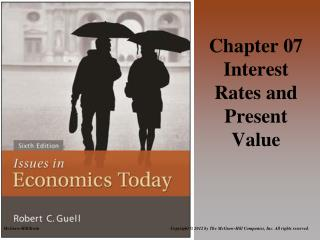DownloadDownload PresentationChapter 07 Interest Rates and Present Value

# Chapter 07 Interest Rates and Present Value

Download Presentation## Chapter 07 Interest Rates and Present Value

- - - - - - - - - - - - - - - - - - - - - - - - - - - E N D - - - - - - - - - - - - - - - - - - - - - - - - - - -
##### Presentation Transcript

1. Chapter 07 Interest Rates and Present Value

2. Chapter Outline • Interest Rates • Present Value • Future Value • Kick It Up a Notch: Risk and Reward

3. The Market for Money Interest Rates

4. Interest Rate • The interest rate is the percentage, usually expressed in annual terms, of a balance that is paid by a borrower to a lender that is in addition to the original amount borrowed or lent.

5. Interest rate (r) Supply r* Demand Money (\$) Borrowed/Saved \$* Figure 1 The Market for Money

6. Nominal vs. Real Interest Rates • Nominal Interest Rate: the advertised rate of interest • Real Interest Rate: the rate of interest after inflation expectations are accounted for; the compensation for waiting on consumption

7. Present Value • Present Value is the interest adjusted value of future payment streams. • Mathematically, the present value of a payment is =(payment)/(1+r)n Where r is the interest rate n is the number of years until the payment is received/made.

8. The Amount Payable for Every Dollar Borrowed (For several interest rates and loan durations)

9. Examples From This Table • If you borrow \$1 and promise to pay it back in 5 years at 5% interest you will owe \$1.28 which is the original \$1 plus 28 cents in interest. • If you borrow \$1 and promise to pay it back in 30 years at 20% interest you will owe \$237.38 which is the original \$1 plus \$236.38 in interest.

10. Mortgages, Car Payments, and other Multiple-Payment Examples • Mortgages are loans taken out to buy homes. Typically you borrow a large sum of money and promise to pay it back in even amounts each month for 10, 15, or 30 years. • Car loans are similar to mortgages in that you borrow a large sum but the loan duration is usually two to six years.

11. A Multiple Year Example @ 5%

12. A Multiple Year Example @ 8%

13. A Multiple Year Example @ 10%

14. Internal rate of return • Internal rate of return : The interest rate where the present value of costs and benefits are equal.

15. Monthly Payments Required on per \$1000 of loan (For Several Interest Rates and Loan Durations)

16. Examples From This Table • If you borrow \$1000 and promise to pay it back monthly over 5 years at 5% interest you will owe \$18.87 per month. • If you borrow \$1000 and promise to pay it back monthly over 10 years at 20% interest you will owe \$19.33 per month.

17. Future Value • Future value: the interest-adjusted value of past payments.

18. Rule of 72 • Rule of 72: A short cut that allows you to estimate the time it would take for an investment to double by dividing 72 by the annual interest rate. • For example: How long would it take to double your money (\$10,000) at 4% interest? • FV formula: \$10,000x(1.04)^18=\$20,258.17 (so a little less than 18 years is the answer). • Rule of 72: 72/4=18 years

19. Risk and Reward Kick It Up A Notch:

20. Kick It Up A Notch: Risk and Reward • Risk: the possibility that the investor will not get those anticipated payoffs • Default Risk: the risk to the investor that the borrower will not pay • Market Risk: the risk that the market value of an asset will change in an unanticipated manner • Reward • Risk Premium the reward investors receive for taking greater risk

21. The Yield Curve • Yield Curve:the relationship between reward and the time until the reward is received US Treasury Yield Curve (January 2005)